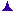3)
2)
4)
1)
5)
6)
7)
8)
9)
10)
11)
12)
13)
14)
15)
16)
17)
18)
19)
20)
21)
22)
23)
24)
25)
26)
27)
28)
29)
30)
31)
32)
33)
34)
35)
36)
37)
38)
39)
40)
41)
42)
43)
44)
45)
46)
47)
48)
49)
50)
51)
52)
1)
2)
3)
4)
Vol. II
Vol. I
Vol. I
Vol. I
Vol. I
Vol. I
Vol. I
5)
6)
7)
8)
9)
10)
Vol. II
11)
12)
13)
14)
15)
16)
17)
18)
19)
Vol. II
20)
21)
22)
23)
24)
25)
26)
27)
28)
29)
30)
Vol. II
31)
32)
33)
34)
35)
36)
37)
38)
39)
40)
41)
42)
43)
44)
45)
46)
47)
48)
49)
50)
Vol. II
Vol. II
51)
52)
Vol. III
1)
2)
3)
4)
5)
6)
7)
8)
9)
10)
Vol. III
11)
12)
13)
14)
15)
16)
17)
18)
19)
20)
Vol. III
21)
22)
23)
24)
25)
26)
27)
28)
29)
30)
Vol. III
31)
32)
33)
34)
35)
36)
37)
38)
39)
40)
Vol. III
41)
42)
43)
44)
45)
46)
47)
48)
49)
50)
Vol. III
51)
52)
1)
Vol. IV
2)
3)
4)
5)
6)
7)
8)
9)
10)
Vol. IV
11)
12)
13)
14)
15)
16)
17)
18)
19)
20)
Vol. IV
21)
22)
23)
24)
25)
26)
27)
28)
29)
30)
Vol. IV
31)
32)
33)
34)
35)
36)
37)
38)
39)
40)
Vol. IV
41)
42)
43)
44)
45)
46)
47)
48)
49)
50)
Vol. IV
51)
52)
1)
Vol.  V
2)
3)
4)
5)
6)
7)
8)
9)
10)
11)
12)
Vol.  V
13)
14)
15)
Vol.  V
16)
17)
18)
19)
20)
21)
Vol.  V
22)
23)
24)
25)
26)
27)
28)
29)
Vol.  V
30)
31)
32)
33)
34)
35)
36)
37)
38)
39)
40)
Vol.  V
41)
42)
43)
44)
45)
46)
47)
48)
49)
50)
Vol.  V
51)
52)
1)
Vol.  VI
Vol.  VI
2)
3)
4)
5)
6)
7)
8)
9)
10)
Vol.  VI
11)
12)
13)
14)
15)
16)
17)
18)
19)
20)
Vol.  VI
21)
22)
23)
24)
25)
26)
27)
28)
29)
30)
Vol.  VI
31)
32)
33)
34)
35)
36)
37)
38)
39)
Vol.  VII
40)
41)
42)
43)
44)
45)
46)
47)
48)
49)
50)
51)
52)
Vol.  VI
1)
2)
3)
4)
5)
6)
7)
8)
9)
10)
Vol.  VII
11)
12)
13)
14)
15)
16)
17)
18)
19)
20)
Vol.  VII
21)
22)
23)
24)
25)
26)
27)
28)
29)
30)
Vol.  VII
31)
32)
33)
34)
35)
36)
37)
38)
39)
40)
Vol.  VII
41)
42)
43)
44)
45)
46)
47)
48)
49)
50)
51)
52)
1)
Vol. VIII
2)
3)
4)
5)
6)
7)
8)
9)
10)
Vol. VIII
11)
12)
13)
14)
15)
16)
17)
18)
19)
20)
Vol. VIII
Vol. VIII
21)
The Sunday Sermons Archive - Index22)
23)
24)
25)
26)
27)
28)
29)
30)
Vol. VIII
31)
32)
33)
34)
35)
36)
37)
38)
39)
40)
Vol. VIII
41)
42)
43)
44)
45)
46)
47)
48)
49)
50)
51)
52)
Vol. XII
Vol. VIII
1)
Vol. IX
2)
3)
4)
5)
6)
7)
8)
9)
10)
Vol. IX
11)
12)
13)
14)
15)
16)
17)
18)
19)
20)
Vol. IX
21)
22)
23)
24)
25)
26)
27)
28)
29)
30)
Vol. IX
31)
32)
33)
34)
35)
36)
37)
38)
39)
40)
Vol. IX
41)
42)
43)
44)
45)
46)
47)
48)
49)
50)
51)
52)
Vol. IX
Vol. IX
1)
2)
3)
4)
Vol.  X
5)
6)
7)
8)
9)
10)
Vol.  X
11)
12)
13)
14)
17)
15)
16)
20)
18)
19)
Vol.  X
21)
23)
22)
24)
25)
26)
27)
28)
29)
31)
Vol.  X
30)
36)
32)
33)
34)
35)
37)
38)
41)
39)
40)
Vol.  X
42)
43)
44)
52)
45)
46)
47)
48)
49)
50)
51)
Vol.  X
1)
2)
3)
4)
5)
6)
7)
8)
9)
10)
Vol. XI
11)
12)
13)
14)
15)
16)
17)
18)
19)
20)
Vol. XI
21)
22)
23)
24)
25)
26)
27)
28)
29)
30)
Vol. XI
31)
32)
33)
34)
35)
36)
37)
38)
39)
40)
Vol. XI
41)
42)
43)
44)
45)
46)
47)
48)
49)
50)
51)
52)
Vol. XI
1)
2)
3)
4)
5)
6)
7)
8)
9)
10)
11)
12)
13)
14)
15)
16)
17)
18)
19)
20)
21)
22)
23)24)
25)26)27)28)29)30)31)
Vol. XII32)33)34)35)36)37)38)39)40)
Vol. XII
Vol. XII41)42)43)44)45)46)47)48)49)50)
Vol. XII51)52)1)
Vol. XIII2)3)4)
Vol. XIII5)6)7)8)9)10)11)12)13)14)15)16)17)18)19)20)21)
Vol. XIII22)23)